# Fundamentals of OFDM Modulation and Demodulation¶

In this notebook, we will introduce the basic OFDM modulation and demodulation in complex baseband and describe the difficulties that arise in a real-world practical implementation.

For a more detailed explanation of the basic OFDM system have a look at the article at DSPIllustrations.com. Basically, one OFDM symbol of length $T$ consists of $K$ subcarriers, where each carrier conveys a single complex-valued QAM symbol. The subcarriers are $1/T$ apart to ensure orthogonality between the carriers. The modulation is usually performed using the inverse Fourier Transform, and at the receiver side an Fourier transform is applied to recover the data.

In addition, a cyclic prefix is added to each symbol in order to combat inter-symbol interference induced by a multipath channel and to transform the linear convolution with the channel's impulse response into a circular convolution.

### OFDM Modulation and Demodulation Equations¶

Mathematically, the OFDM transmit signal is obtained as follows. We define the QAM symbols $X$ in the frequency domain. Then, the time-domain transmit signal $x[n]$ is obtained by

$$x[n] = \sum_{k=0}^{K-1} X[k]\exp(j2\pi kn/K),\quad n=-N_{CP},\dots, K-1$$

By taking a closer look, we see that the summation is actually a discrete Fourier transform, and hence we can write

$$x[n] = K\cdot \text{IDFT}_K\{X[k]\}[n], \quad n=0,\dots,K-1$$

Afterwards, a CP is added to the signal.

At the receiver side the inverse operation is performed to retrieve the transmitted symbols:

$$\hat{X}[k] = \sum_{n=0}^{N-1}x[n]\exp(-j2\pi kn/K)$$

Taking a closer look, above expression is equal to a Discrete Fourier Transform and hence

$$\hat{X}[k] = \text{DFT}_K\{x[n]\}[k]$$

If we now insert the expression for the transmitted signal we get

$$\hat{X}[k] = \text{DFT}_K\{K\cdot\text{IDFT}_K\{X[k]\}[n]\}[k] =K X[k]$$

which shows that the received data equals the transmitted data up to a scaling factor.

### Effect of a multipath channel¶

Consider now, that the transmitted signal is sent through a multipath channel with impulse response $h[n]$. At the receiver side we have

$$\hat{X}[k] = K\cdot \text{DFT}_K\{h[n]*\text{IDFT}_K\{X[k]\}[n]\}[k]$$

Now, using the convolution theorem (convolution in time is multiplication in frequency domain), this expression leads to

$$\hat{X}[k] = K\cdot \text{DFT}_K \{\text{IDFT}_K\{H[k]X[k]\} \} = K H[k]X[k]$$

where $H[k]$ is the DFT of the impulse response. Note that above derivation only works for a sufficiently long CP that transform the linear convolution of the channel into a circular convolution.

Hence, what we see is that the received symbols are equal to the transmitted symbols multiplied by the frequency response of the channel. There is no interference and we can use a simple one-tap equalizer.

## Implementation of the baseband OFDM Tx and Rx¶

Now that we have talked about the fundamentals, let's start implementing the modulation and demodulation:

In :
from audioComms.components import Component, TX, Environment, Recorder
from audioComms.utils import StatefulFilter, get_filter
from audioComms.plotting import PlotWaveform, PlotSpectrum, PlotConstellation, PlotBlock
from audioComms.channels import AudioChannel, SimulatedChannel, IdentityChannelEffect, SimulatedChannelEffect, NoiseChannelEffect


To not become overwhelmed by the number of parameters, we combine all OFDM-related parameters into a structure:

In :
# The OFDM parameter structure describing the OFDM symbol
class OFDM:
def __init__(self, **kwargs):
for k, v in kwargs.items():
setattr(self, k, v)
ofdm = OFDM()
ofdm.K = 256                      # Number of OFDM subcarriers
ofdm.Kon = 200                    # Number of switched-on subcarriers
ofdm.CP = 64                      # Number of samples in the CP


Here, you might wonder what the parameter Kon means. It's the number of subcarriers that actually carry data. In practice, guard bands are left at both sides of the OFDM spectrum, such that not the full spectrum is used for data transmission. The used carriers are located around the DC frequency, whereas the guards appear at the edge of the band. We will shortly, what this means.

First, let's focus on the modulation. To modulate some data, we need to have a random data generator:

In :
def random_qam(ofdm):
qam = np.array([1+1j, 1-1j, -1+1j, -1-1j]) / np.sqrt(2)
return np.random.choice(qam, size=(ofdm.Kon), replace=True)

random_qam(OFDM(K=8, Kon=4))

Out:
array([ 0.70710678-0.70710678j, -0.70710678+0.70710678j,
0.70710678-0.70710678j,  0.70710678-0.70710678j])

The random_qam function creates random QPSK symbols that will be modulated into an OFDM symbol. We are now ready to actually write the OFDM modulation function:

In :
# Source code has been redacted in online version
# Omitting 10 lines of source code


Let's run this function with some example data and look at the spectrum of the output:

In :
ofdm = OFDM(K=64, Kon=21, CP=16)
tx = ofdm_modulate(ofdm, random_qam(ofdm))
f = np.linspace(-ofdm.K/2, ofdm.K/2, len(tx)*8, endpoint=False)

plt.figure(figsize=(8,3))
plt.plot(f, abs(np.fft.fftshift(np.fft.fft(tx, 8*len(tx)))))
plt.grid(True); plt.xlabel('Subcarrier index'); plt.ylabel('|X(f)|')

plt.gca().add_patch(patches.Rectangle((-10,0), 20, 20, linewidth=1, fill=None, hatch='/'));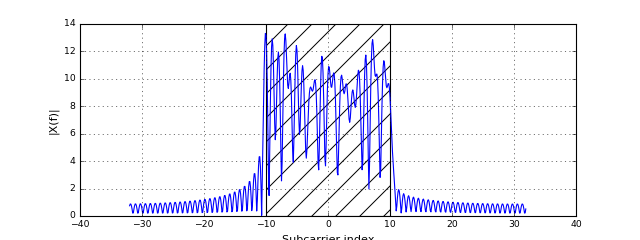Ok, we allocated 21 out of 64 subcarriers. Looking at the spectrum of the modulated signal we see that the carriers around DC are allocated, ranging from carrier with index $k=-10$ to carrier with index $k=+10$. Note, that the carrier with index $k=-10$ is actually the carrier with index $k=K-10=54$ when considering the modulation equation from above.

Now, given this basic OFDM modulation, we can write the component that creates the signal that is used for our audio transmission:

In :
class OFDMTransmitter(TX):
def __init__(self, environment, ofdm):
super().__init__(environment)
self._ofdm = ofdm

def _generateSignal(self):
qam = random_qam(self._ofdm)              # Create random data
sym = ofdm_modulate(self._ofdm, qam)      # Perform OFDM modulation
return sym


As this component is straight-forward, let's use it in a simple simulation: Note that here we cannot yet use the Audio channel as we are still operating an complex-valued baseband which cannot be transmitted in reality. Later on, we will extend the system to use the up- and downconversion for a real audio transmission.

In :
def runTransmission():
env = Environment(samplerate=1000)
ofdm = OFDM(K=256, Kon=128, CP=64)

tx = OFDMTransmitter(env, ofdm)
channel = SimulatedChannel(env)
fig = env.figure(figsize=(10,3))
showWave = PlotWaveform(env, windowDuration=256+64, integerXaxis=True, isComplex=True, axes=fig.add_subplot(121), ylim=(-2,2))
showSpec = PlotSpectrum(env, windowDuration=0.5, axes=fig.add_subplot(122), logScale=True)

tx.transmitsTo(channel)
channel.transmitsTo(showWave)
channel.transmitsTo(showSpec)

env.run()
runTransmission()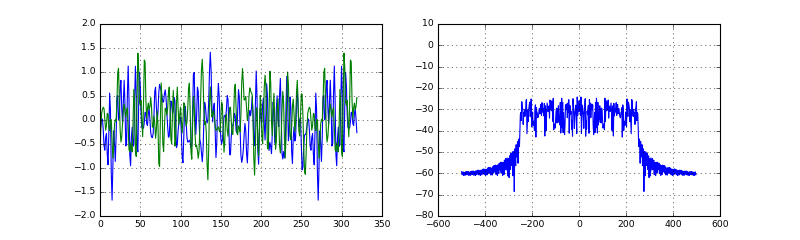Stop received from external


OK, the modulator is running, and constantly generating data. Now, let's go on and write the most basic OFDM receiver: This receiver assumes a continuous stream of OFDM symbols is received, and it cuts out CP for every symbol and forwards the extracted symbol to the demodulator. Due to the streaming nature with random signal lengths we need to do some extra work to keep track of the frame extraction:

In :
# Source code has been redacted in online version
# Omitting 31 lines of source code


Let us try this receiver in the most basic channel, a simple AWGN channel. Again, we cannot use the audio channel yet ase we are still in baseband.

In :
# Source code has been redacted in online version
# Omitting 15 lines of source code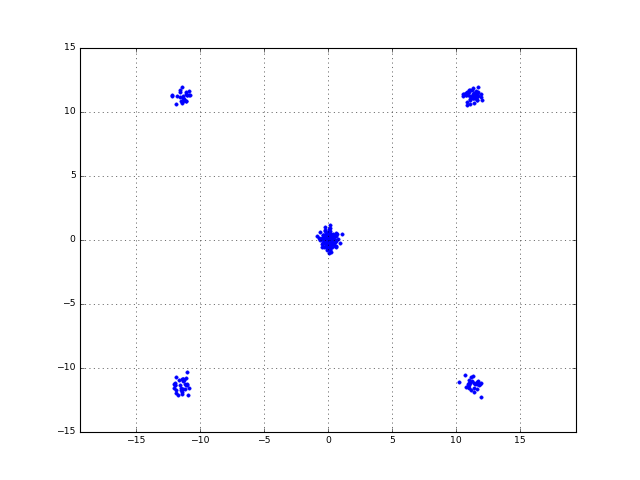Stop received from external


Great! We can clearly see the QPSK constellation. In addition, we see the zero-values which correspond to the non-allocated subcarriers. As you can see, the most basic OFDM receiver does not cut these samples away.

## Moving to Passband¶

As the OFDM signal is a complex-valued signal, we need to transform it into the passband to a certain carrier frequency such that we can transmit it over a real channel. As we have already prepared the up- and downconversion components it is simply a matter of plugging them together to reach our goal:

In :
from audioComms.passband import InterpolationUpconversion, DownconversionDecimation


Let us just include the up- and downconversion and see how the system works:

In :
def runTransmission(channelFunc):
env = Environment(samplerate=44100)
ofdm = OFDM(K=256, Kon=128, CP=64)
B = 5*441     # Baseband bandwidth in Hz
Fc = 10000    # carrier frequency in Hz

tx = OFDMTransmitter(env, ofdm)
upc = InterpolationUpconversion(env, B=B, Fc=Fc)
channel = channelFunc(env)
down = DownconversionDecimation(env, B=B, Fc=Fc)
constellation = PlotConstellation(env)

tx.transmitsTo(upc)
upc.transmitsTo(channel)
channel.transmitsTo(down)
down.transmitsTo(rx)
rx.transmitsTo(constellation)

env.run()
runTransmission(SimulatedChannel)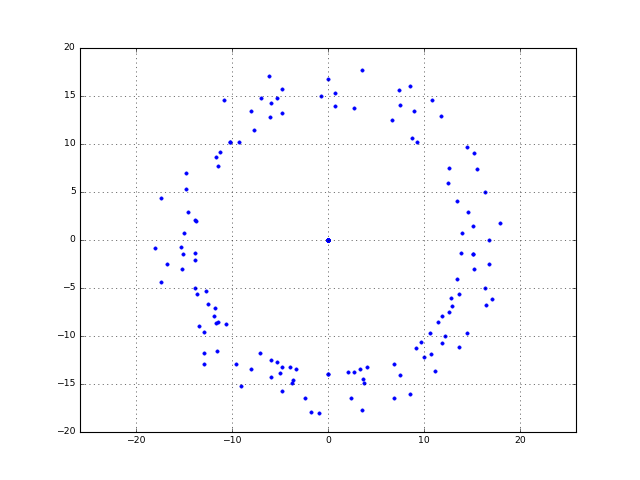Stop received from external


Uh... what's going on here? Where is the constellation gone? Why do we see a circle instead of clear constellation points? The answer is: The up- and downconversion operations introduce some delay which is not accounted for in the receiver. The receiver naively assumes that the first received sample belongs to the CP, and some samples later the actual symbol starts. it does not consider (and it cannot) any information where the OFDM symbol really starts. Since we know (check the definition of UpDownConversionFilter from the previous notebook) that both the up- and downconversion introduce a delay of 3 baseband samples each, we can manually compensate for this:

In :
from audioComms.channels import InitialLossChannelEffect

runTransmission(lambda env: SimulatedChannel(env, InitialLossChannelEffect(int((3+3)*44100/(5*441)))))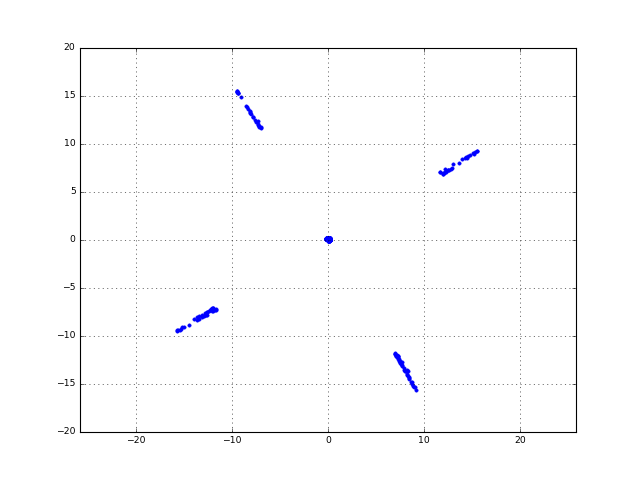Stop received from external


OK, that's much better. We can now clearly see some constellation points. However, they seem a bit rotated. In addition, the points do not lie on one spot, but form lines at each constellation point. The reason can be found by the up- and downconversion: As we have seen in the previous notebook, the up- and downconversion can introduce a phase shift based on the occuring delay. This phase shift is reflected by the rotation of the constellation diagram. Moreover, the line-shapes of the received constellation points are due to the truncation of the interpolation filter used for up- and downconversion. With a more accurate filter (with longer delay), these effects could be reduced. However, in the following notebooks we will find ways to mitigate these problems by signal processing at the receiver side, namely synchronization and channel estimation.

## Using the real Audio channel¶

Up to now, we only used the deterministic simulated channel. Now, let's try with the system with using the audio signal:

In :
runTransmission(AudioChannel)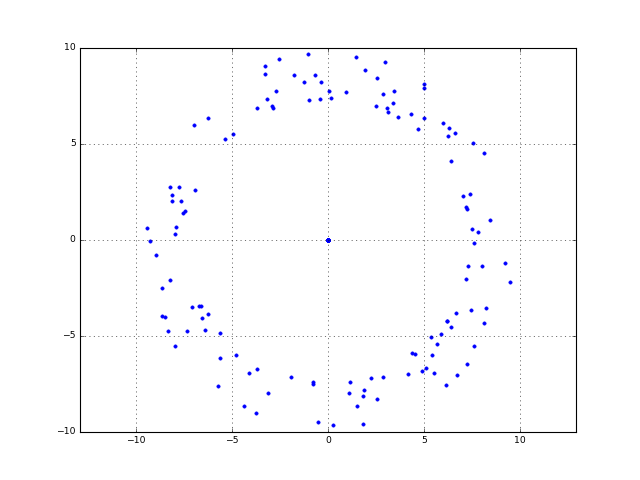Stop received from external


In the audio channel, the constellation set switches between the circle-like and complete noise constellations. If you are really lucky, you will see a constellation, but this is very unlikely. The audio channel does not introduce a deterministic delay. Instead depending on the microphone position and internal buffers etc. the delay can be changing over time. Hence we will rarely see a constellation diagram.

## Conclusion¶

In this notebook, we have developed a simple OFDM transmitter and most basic receiver. We have seen that random delay in the channel hinders the receiver to extract the correct symbols from the received signal and hence cannot obtain the transmitted constellation.

What we need is a method to automatically determine the starting point of the OFDM symbols. This, so-called synchronization will be the focus of the upcoming notebook.

DSPIllustrations.com is a participant in the Amazon Services LLC Associates Program, an affiliate advertising program designed to provide a means for sites to earn advertising fees by advertising and linking to amazon.com, amazon.de, amazon.co.uk, amazon.it.